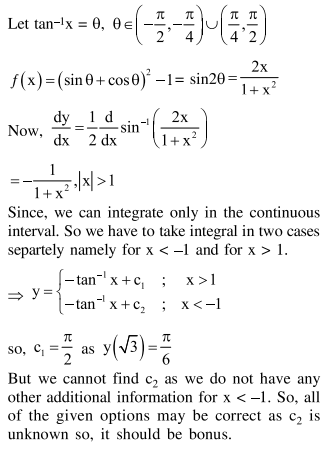# Solve this following

Question:

Let $f(x)=\left(\sin \left(\tan ^{-1} x\right)+\sin \left(\cot ^{-1} x\right)\right)^{2}-1,|x|>1$.

If $\frac{\mathrm{dy}}{\mathrm{dx}}=\frac{1}{2} \frac{\mathrm{d}}{\mathrm{dx}}\left(\sin ^{-1}(f(\mathrm{x}))\right)$ and $\mathrm{y}(\sqrt{3})=\frac{\pi}{6}$, then $\mathrm{y}(-\sqrt{3})$ is equal to

1. $\frac{5 \pi}{6}$

2. $-\frac{\pi}{6}$

3. $\frac{\pi}{3}$

4. $\frac{2 \pi}{3}$

Correct Option: 1

Solution: